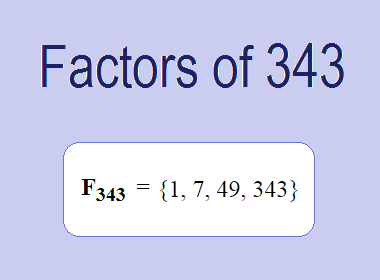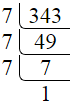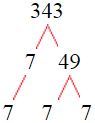# Factors of 343The factors of 343 are 1, 7, 49, and 343 i.e. F343 = {1, 7, 49, 343}. The factors of 343 are all the numbers that can divide 343 without leaving a remainder.

We can check if these numbers are factors of 343 by dividing 343 by each of them. If the result is a whole number, then the number is a factor of 343. Let's do this for each of the numbers listed above:

·        1 is a factor of 343 because 343 divided by 1 is 343.

·        7 is a factor of 343 because 343 divided by 7 is 49.

·        49 is a factor of 343 because 343 divided by 49 is 7.

·        343 is a factor of 343 because 343 divided by 343 is 1.

## How to Find Factors of 343?

1 and the number itself are the factors of every number. So, 1 and 343 are two factors of 343. To find the other factors of 343, we can start by dividing 343 by the numbers between 1 and 343. If we divide 343 by 2, we get a remainder of 1. Therefore, 2 is not a factor of 343. If we divide 343 by 3, we get a remainder of 1. Therefore, 3 is not a factor of 343.

Next, we can check if 4 is a factor of 343. If we divide 343 by 4, we get a remainder of 3. Therefore, 4 is not a factor of 343. Similarly, If we divide 343 by 7, we get a remainder of 0. Therefore, 7 is a factor of 343. We can continue this process for all the possible factors of 343.

Through this process, we can find that the factors of 343 are 1, 7, 49, and 343. These are the only numbers that can divide 343 without leaving a remainder.

********************

********************

********************

## Properties of the Factors of 343

The factors of 343 have some interesting properties. One of the properties is that the sum of the factors of 343 is equal to 400. We can see this by adding all the factors of 343 together:

1 + 7 + 49 + 343 = 400

Another property of the factors of 343 is that they are all odd numbers. This is because 343 is an odd number, and any even number cannot divide an odd number without leaving a remainder.

Another property of the factors of 343 is that the only prime factor of 343 is 7.

## Applications of the Factors of 343

The factors of 343 have several applications in mathematics. One of the applications is in finding the highest common factor (HCF) of two or more numbers. The HCF is the largest factor that two or more numbers have in common. For example, to find the HCF of 343 and 21, we need to find the factors of both numbers and identify the largest factor they have in common. The factors of 343 are 1, 7, 49, and 343. The factors of 21 are 1, 3, 7, and 21. The largest factor that they have in common is 7. Therefore, the HCF of 343 and 21 is 7.

Another application of the factors of 343 is in prime factorization. Prime factorization is the process of expressing a number as the product of its prime factors. The only prime factor of 343 is 7. We can express 343 as:

343 = 7 × 7 × 7

We can do prime factorization by division and factor tree method also. Here is the prime factorization of 343 by division method,343 = 7 × 7 × 7

Here is the prime factorization of 343 by the factor tree method,343 = 7 × 7 × 7

## Conclusion

The factors of 343 are the numbers that can divide 343 without leaving a remainder. The factors of 343 are 1, 7, 49, and 343. The factors of 343 have some interesting properties, such as being odd numbers and having a sum of 400. The factors of 343 have several applications in mathematics, such as finding the highest common factor and prime factorization.